# Energy equation

The energy equation is given, in classical thermodynamics, by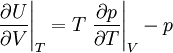$\left. \frac{\partial U}{\partial V} \right\vert_T = T \left. \frac{\partial p}{\partial T} \right\vert_V -p$

and in statistical mechanics it is obtained via the thermodynamic relation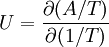$U = \frac{\partial (A/T)}{\partial (1/T)}$

and making use of the Helmholtz energy function and the canonical partition function one arrives at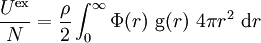$\frac{U^{\rm ex}}{N}= \frac{\rho}{2} \int_0^{\infty} \Phi(r)~{\rm g}(r)~4 \pi r^2~{\rm d}r$

where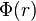$\Phi(r)$ is a two-body central potential,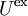$U^{\rm ex}$ is the excess internal energy per particle, and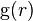${\rm g}(r)$ is the radial distribution function.